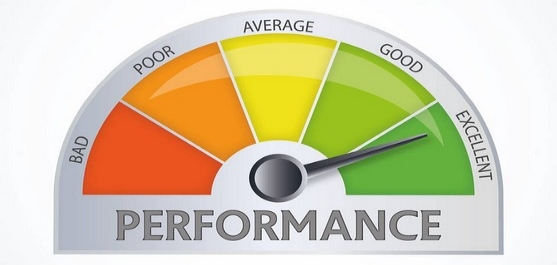## Kaggle Evaluation Metrics Used for Regression Problems

2019-02-16"This post describe evaluation metrics used in Kaggle competitions where problem to solve is has regression nature. Eight different metrics are described, namely - Absolute Error (AE), Mean Absolute Error (MAE), Weighted Mean Absolute Error (WMAE), Pearson Correlation Coefficient, Spearman\u2019s Rank Correlation, Root Mean Squared Error (RMSE), Root Mean Squared Logarithmic Error (RMSLE), Mean Columnwise Root Mean Squared Error (MCRMSE)."